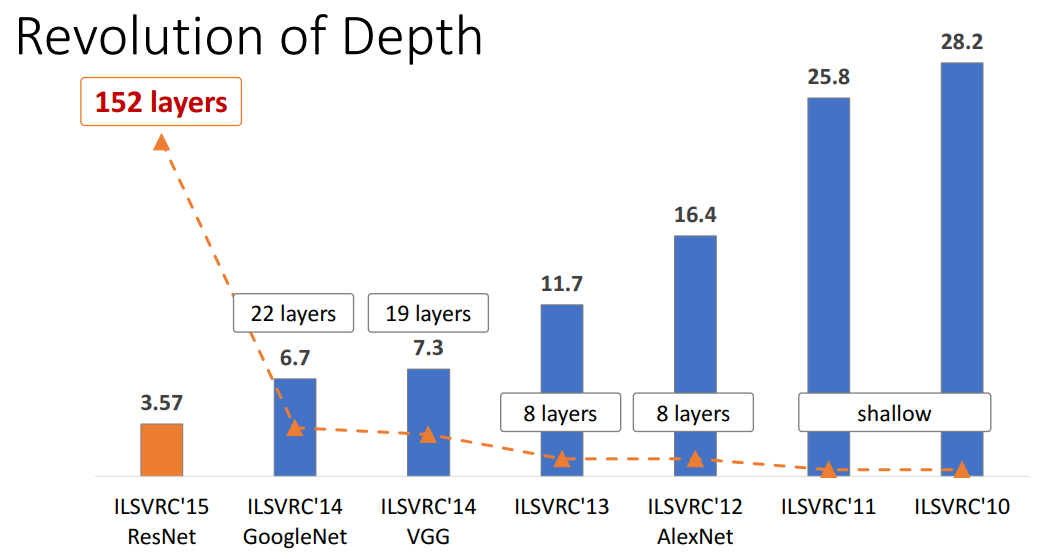## 引言

ResNet取得了5项第一，并又一次刷新了CNN模型在ImageNet上的历史：

ResNet的作者何凯明也因此摘得CVPR2016最佳论文奖，当然何博士的成就远不止于此，感兴趣的可以去搜一下他后来的辉煌战绩。那么ResNet为什么会有如此优异的表现呢？其实ResNet是解决了深度CNN模型难训练的问题，从图2中可以看到14年的VGG才19层，而15年的ResNet多达152层，这在网络深度完全不是一个量级上，所以如果是第一眼看这个图的话，肯定会觉得ResNet是靠深度取胜。事实当然是这样，但是ResNet还有架构上的trick，这才使得网络的深度发挥出作用，这个trick就是残差学习（Residual learning）。下面详细讲述ResNet的理论及实现。

## 残差学习## ResNet的网络结构

ResNet网络是参考了VGG19网络，在其基础上进行了修改，并通过短路机制加入了残差单元，如图5所示。变化主要体现在ResNet直接使用stride=2的卷积做下采样，并且用global average pool层替换了全连接层。ResNet的一个重要设计原则是：当feature map大小降低一半时，feature map的数量增加一倍，这保持了网络层的复杂度。从图5中可以看到，ResNet相比普通网络每两层间增加了短路机制，这就形成了残差学习，其中虚线表示feature map数量发生了改变。图5展示的34-layer的ResNet，还可以构建更深的网络如表1所示。从表中可以看到，对于18-layer和34-layer的ResNet，其进行的两层间的残差学习，当网络更深时，其进行的是三层间的残差学习，三层卷积核分别是1x1，3x3和1x1，一个值得注意的是隐含层的feature map数量是比较小的，并且是输出feature map数量的1/4。

## ResNet的TensorFlow实现

class ResNet50(object):
def __init__(self, inputs, num_classes=1000, is_training=True,
scope="resnet50"):
self.inputs =inputs
self.is_training = is_training
self.num_classes = num_classes

with tf.variable_scope(scope):
# construct the model
net = conv2d(inputs, 64, 7, 2, scope="conv1") # -> [batch, 112, 112, 64]
net = tf.nn.relu(batch_norm(net, is_training=self.is_training, scope="bn1"))
net = max_pool(net, 3, 2, scope="maxpool1")  # -> [batch, 56, 56, 64]
net = self._block(net, 256, 3, init_stride=1, is_training=self.is_training,
scope="block2")           # -> [batch, 56, 56, 256]
net = self._block(net, 512, 4, is_training=self.is_training, scope="block3")
# -> [batch, 28, 28, 512]
net = self._block(net, 1024, 6, is_training=self.is_training, scope="block4")
# -> [batch, 14, 14, 1024]
net = self._block(net, 2048, 3, is_training=self.is_training, scope="block5")
# -> [batch, 7, 7, 2048]
net = avg_pool(net, 7, scope="avgpool5")    # -> [batch, 1, 1, 2048]
net = tf.squeeze(net, [1, 2], name="SpatialSqueeze") # -> [batch, 2048]
self.logits = fc(net, self.num_classes, "fc6")       # -> [batch, num_classes]
self.predictions = tf.nn.softmax(self.logits)

def _block(self, x, n_out, n, init_stride=2, is_training=True, scope="block"):
with tf.variable_scope(scope):
h_out = n_out // 4
out = self._bottleneck(x, h_out, n_out, stride=init_stride,
is_training=is_training, scope="bottlencek1")
for i in range(1, n):
out = self._bottleneck(out, h_out, n_out, is_training=is_training,
scope=("bottlencek%s" % (i + 1)))
return out

def _bottleneck(self, x, h_out, n_out, stride=None, is_training=True, scope="bottleneck"):
""" A residual bottleneck unit"""
n_in = x.get_shape()[-1]
if stride is None:
stride = 1 if n_in == n_out else 2

with tf.variable_scope(scope):
h = conv2d(x, h_out, 1, stride=stride, scope="conv_1")
h = batch_norm(h, is_training=is_training, scope="bn_1")
h = tf.nn.relu(h)
h = conv2d(h, h_out, 3, stride=1, scope="conv_2")
h = batch_norm(h, is_training=is_training, scope="bn_2")
h = tf.nn.relu(h)
h = conv2d(h, n_out, 1, stride=1, scope="conv_3")
h = batch_norm(h, is_training=is_training, scope="bn_3")

if n_in != n_out:
shortcut = conv2d(x, n_out, 1, stride=stride, scope="conv_4")
shortcut = batch_norm(shortcut, is_training=is_training, scope="bn_4")
else:
shortcut = x
return tf.nn.relu(shortcut + h)

## 总结

ResNet通过残差学习解决了深度网络的退化问题，让我们可以训练出更深的网络，这称得上是深度网络的一个历史大突破吧。也许不久会有更好的方式来训练更深的网络，让我们一起期待吧！